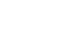Standard error (often shortened to SE) is the estimated standard deviation of the sampling distribution of a parameter. It is a way of quantifying the uncertainty around a parameter that has been estimated from some data.

## Why are standard errors important?

The uncertainty of a parameter estimate is important in determining how we should interpret the estimate. For example, suppose we measured the heights of people in samples from populations A and B and we computed the means from each sample and found that the mean from sample A was less than the mean of sample B. We might therefore jump to the conclusion that the people in population A are shorter than the people in population B. This is not necessarily true. If there is a sufficient amount of uncertainty around the means and we were to perform the same comparison on different samples, it would be possible to get the opposite result - where sample A has a higher mean than sample B. Therefore, any comparison of the means without knowing their uncertainty is meaningless.

If we knew the standard errors of the means, we could use them to perform a two-sample t-test comparing two means, which would test against the null hypothesis that the mean is zero. Describing this test is beyond the scope of this article, but essentially it is able to tell us whether population A is shorter than population B or vice versa, up to a certain level of confidence.

## How do I compute a standard error?

There is no single formula for the standard error, since the method of estimating the standard deviation of a parameter depends on the parameter itself. Perhaps the simplest and most widely-known case is the standard error of the mean of a sample. This is simply the sample standard deviation (SD) divided by the square root of the sample size (n):This formula tells us that the standard error of the mean is smaller when the sample size is larger (this is true for standard errors in general). However, because we are dividing by the square root of the sample size, increasing the sample size has a diminishing effect on reducing the standard error.

In other simple problems such as ordinary least squares, a formula for the standard error of regression coefficients also exists. For more complicated parameters, the only option is to use bootstrapping. Bootstrapping works by estimating the parameter from a data set produced by sampling the original data with replacement. This process is repeated multiple times, so that a different estimate is produced each time. The bootstrapped standard error is simply the standard deviation of these parameter estimates. With sufficient bootstrap samples, this method produces a surprisingly good estimate of the standard error. One drawback is it can be a computationally expensive option if parameter estimation is already slow, because the parameter will need to be estimated for each bootstrap sample.

Find out more with our Beginner's Guides!